A Reintroduction of Epicycles: Newton's 1702 Lunar Theory and Halley's Saros Correction

N. Kollerstrom

Q. J. R. astr. Soc. (1995), 36, 357-368

This essay was given as an address to the RAS in May 1994. It summarizes arguments in the author’s PhD thesis `The Achievement of Newton's 1702 Theory of the Moon's Motion' 1995. For a fuller account, see the author’s ‘Newton’s Forgotten Lunar Theory’ 2000, (www.greenlion.com/Roar2000.html) recently described by James Gleick as ‘definitive:’ ‘Isaac Newton,’ 2003, p.238)

1 BACKGROUND

Three hundred years ago, in 1694, Isaac Newton paid a visit to Britain's only professional astronomer, the Reverend John Flamsteed, in his newly ­established Royal Observatory at Greenwich (1). He was there shown a table of lunar longitude observations, plus a column of associated error values, as differences between observed longitudes and corresponding values Flamsteed had derived from a lunar theory. These error values were generally within eight arcminutes (2).

These error-values became the stimulus for Newton's great endeavour to resolve the erratic motions of the Moon. The prediction of longitude at sea was the most pressing scientific problem of the age, and the finding of lunar longitude seemed to be a promising approach : it gave a version of universal time, from the Moon's position, which could be compared with local time, whereby longitude could be inferred (3).

The lunar method used and published by Flamsteed consisted of three steps or `equations' and was derived from Jeremiah Horrox (4). As the Astronomer Royal, Flamsteed had come to despair of being able to find longitude by using this approach, even though it was the primary reason for the setting up of the Observatory at Greenwich in 1676. In 1683 he had published an article in the Philosophical Transactions advocating use of the positions of the moons of Jupiter as a more reliable method (5).

Britain's first lunar theory had been formed in the 163os, by Horrox in the North of England. It worked kinematically, having a deferent-wheel that revolved once per conjunction of the Sun and the mean apse line (6). It combined a varying eccentricity with an oscillating apse line, both linked to the Sun-apse angle. As the position of perigee rocks backwards and forwards twice-yearly, so astronomers following Horrox likewise envisaged the apse line as rocking to and fro 12 degrees, on top of its gradual forward motion (7). It performed this oscillation twice per 13 months, that being the period of a solar revolution against the mean apse. We may note that modern lunar theory has nothing resembling this rocking of the apse line (8).

As Newton observed to Flamsteed in 1695, the Horroxian theory generated lunar longitude errors of up to at least 12 arcmin, which was far from adequate (9). For comparison, the prizes offered in the next century for `finding the longitude' began at one degree of terrestrial longitude, which is equivalent to some two minutes of lunar longitude (10). A lunar theory had to start by fixing five mean motions, for the Sun, Moon, lunar node, lunar apse and aphelion, as linearly time-dependent functions moving around the ecliptic. These then became `equated' in a series of steps. An `equation' did not then signify a formula, but rather a quantity, measured in minutes or degrees, whereby a mean value was improved, to obtain something nearer to its actual value. For the lunar nodes, Kepler and Brahe had found just one such equation. The Sun likewise was given one `equation' by astronomers, namely its equation of centre.

In the case of the Moon, from Ptolemy to Copernicus there had remained two such `equations', namely the evection and equation of centre; two more had been added by Kepler and Tycho Brahe, namely the annual equation and variation. The key feature of Horrox's theory was its compression of the two classical inequalities into one, which caused both the eccentricity and apse line to co-vary, resulting in a three-stage process (ii): first, the annual equation, then second, the equation of centre and third, the variation.

On Flamsteed's version of the Horroxian theory, the annual equation of the Moon had a maximal amplitude of 12 arcminutes, the equation of centre 6°2o', and the variation 38 arcminutes. Thus the second was far the largest `equation'. Finally, from the node's equated position one obtained the `reduction', to convert longitude positions from the plane of the lunar orbit to that of the ecliptic.

2 GRAVITY THEORY

Whiteside has described how Newton tried and failed in 1694/5 to infer the lunar inequalities from his theory of gravity, whereby his initial optimism gave way to frustration (12). The first edition of the Principia (1687) had contained nothing of assistance to the practical astronomer in this respect. It credibly linked the two inequalities discovered by Kepler and Brahe, namely the annual equation and variation, to the new theory of gravity, and it claimed that the rocking apse line could be likewise accounted for by that theory, however it lacked what was most desired, namely a procedure for locating lunar latitude and longitude. The all-important 'Moon-test' in the First Edition which linked celestial and terrestrial physics used only simple uniform circular motion, with the Earth at the centre (13).

A myth belonging to the 1690s which has rolled down the centuries is the notion that Newton abandoned his lunar endeavour because Flamsteed could not or would not provide him with adequate lunar data (14). The truth is rather the contrary, that Newton had been supplied with an unprecedented amount of positional data - nigh on 200 lunar positions - of a higher accuracy than any hitherto obtained (15). It was rather the inherent difficulty of the problem that caused this failure, if such it was.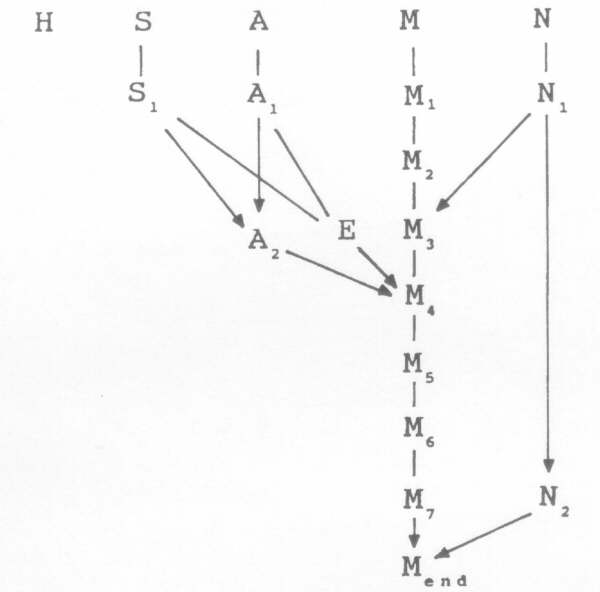FIG. I. The steps of equation given to the mean motions of aphelion, Sun, lunar apse, Moon and lunar node, as H, S, A, M and N, in Newton's 1702 lunar theory.

In the year 1702 Newton's four-page `Theory of the Moon's Motion' (TMM) was published, in a mathematical-astronomical textbook published by David Gregory, who gave it its title (16). My researches did not confirm that Newton had composed this opus in the 169os and merely held it back until then, as some have suggested. Yet, considering that Newton was in 1702 fully occupied as the new Master of the Mint in London, this was a surprising moment to produce a lunar theory as the fruit of earlier labours.

TMM featured six new `equations', such that the Moon became equated seven times. Its procedure is given as a flow diagram in Fig. I, where the symbols used are S for Sun, M: Moon, A: apse, N: node and H: aphelion. The method developed by Newton was essentially Horroxian, though somewhat refined (17). The new lunar equations are the second, third, sixth and seventh, with the annual equation as the first and the variation as the fifth. Also the node and apse line were given `annual equations', making them vary seasonally. A Horroxian equation of centre forms the central fourth `equation' of TMM, using the varying eccentricity E and the twice­ equated apse A2.

The Newtonian `theory' of 1702 contained nothing resembling calculus, nor had it any inherent connection with gravity theory, which was unmentioned in the text. One may query whether it contained trigonometric time-dependent functions: the instructions were all in words, thus it would specify that what was in fact a sine function should be positive up to I8o° and then be made negative after that - readers could evidently not take for granted that a sine function would turn negative above that value.

3 A MODERN RECONSTRUCTION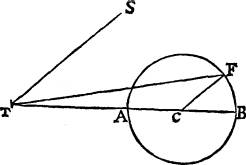FIG. 2. The Horroxian epicycle in Newton's 1702 lunar theory, where T is Earth's centre, S that of the Sun, TB the mean apse, C the mean centre of the lunar orbit and F the `equated' centre of the lunar orbit. TF represents the varying eccentricity and angle FTC the second apse equation. The line CF revolves once per Sun-apse line conjunction.

A computer program was written on a spreadsheet to model these steps of the equation. The `equations' are normally represented by sine and cosine functions; for example the variation is represented by 35'23" sin 2(Sr M4), an expression which reaches its maxima and minima in the octants. The term M4 means that the fourth-equated value of lunar longitude is used. It has been subtracted from the first-equated value of solar longitude, S1. However, TMM also specified that the maximum amplitude of the variation itself varied seasonally, becoming greatest at perihelion. It was to vary `reciprocally as the Cube of the Distance of the Sun from the Earth'. Accordingly, TMM's fifth lunar equation was represented by:

M (5)=M(4), + 35'23" [1-3Ecos (H- S)] sin 2(S1 M4)

where E is the Earth's eccentricity (18). The seven steps of equation for the Moon, but without their amplitude-modulating functions, have been written out in Table I, below.

To the mean apse, TMM added first an annual equation, here modelled by 20'sin(H- S), which maximizes at the equinoxes. This gave A1, the first-­equated apse value. The second equation of the apse was far larger and varied with the Sun's angle against this mean apse, adding which transformed A1 into A2. We may write, A2 = A1 +f(S,-A1), where f is a function using the cosine rule derived from the Newtonian instructions, as a trigonometric equivalent. Fig. 2 shows TMM's diagram of this second apse equation.

An `equation of centre' signified the divergence from uniform circular motion due to elliptical motion according to Kepler's second law. It had zero magnitude at the apogee/perigee positions and for the lunar orbit reached its maximum value of around seven degrees near the quadratures. Its solution involved approximations to the ` Kepler equation', or some other method for those astronomers who had not accepted Kepler's second law.

In constructing the computer replica of TMM, there was only one stage where a modern formula was used in place of the historic procedure or a model thereof. It was ascertained by Gingerich and Welther at the Smithsonian Institute, that Flamsteed's `Equation of Centre' tables, as published in 1681, concurred with values derived using the modern equation of centre formula within several arcseconds (19). Flamsteed was, they concluded, the first to prepare such tables based upon a sufficiently exactsolution of Kepler's area law. Newton's TMM of 1702 merely indicated that tables should be found for `the Equation of the Moon's Centre, as in the common way' (20). Accordingly, the TMM program used the modern equation of centre (21), with input as the anomaly [A(2),-M(3)] and the eccentricity E.

4 ESTIMATES OF ACCURACY

The sign of TMM's sixth equation was given the wrong way round in the 1702 version, and not corrected until 1713 in the Principia's second edition. As this term has a maximum value of two and a half minutes, a wrong sign would generate up to five arcmin of error. It was a vital matter for the astronomers who adopted Newton's seven-step procedure in the first half of the eighteenth century, to see that they had applied it correctly (22).

Edmond Halley and Flamsteed maintained widely diverging views on the accuracy of Newton's lunar theory; the former claimed it was usually accurate to 2-3 arcmin (23) while the latter found errors of 8 or more arcmin in its predictions (24). Its accuracy has here been ascertained by comparing results generated by the computer replica of TMM with positions given by the Improved Linear Ephemeris program (supplied by Bernard Yallop of the RGO) (25). Starting from noon GMT I68o December 31st, longitude positions were generated at daily intervals using (a) the TMM program and (b) the modern (I.L.E.) program for the same times, then the two were subtracted. The differences were plotted as an error curve, giving Fig. 3 as a definitive answer to the accuracy of Newton's lunar theory. The thin line represents the 1702 version with the sixth equation the wrong way round, and the thick line is the corrected version. The standard deviation of the corrected version was I.9 arcminutes.

Textbooks using the TMM procedure normally contained one or two worked examples. These I found tended to show larger errors than those of the computer replica of TMM. Taking 15 such worked examples from eight astronomy books of the period (allowing two per textbook), their mean error in predicted lunar longitude (as the difference, historical estimate - modern ILE value) was found to be -2'.7±4'.0 and these books are listed in an Appendix. Such larger errors may have resulted from: equation of centre errors, inappropriate application of the new equations and interpolation errors in reading the tables. However the four worked examples given in the two latest TMM-based textbooks, of Lemonnier (1746) and Halley (1749), showed errors in lunar longitude between one and two arcminutes, which accords better with our error-estimate.

ERROR PATTERN OF TMM

daily noon values GMT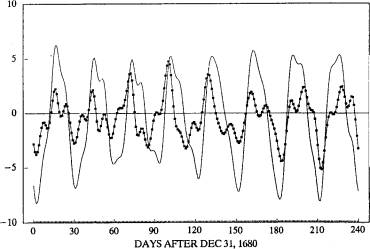- 6TH EQUATION REVERSED

FIG. 3. Comparison of daily error-values of original 1702 version of TMM (thin line) with that obtained after reversing the sixth equation (dotted line), sampling over 240 days.

5 COMPARISON WITH MODERN TERMS

It is of interest to compare the Newtonian terms with the modern ones, even though there is a major obstacle in that the two operational sequences function quite differently: the Newtonian terms are `equated' at every stage, starting from the mean motion and ending with the answer, whereas the modern terms use the same mean values, and the answer is not given at the end, but is obtained by summing the arguments of each equation plus the mean longitude. Table I compares the TMM terms with the first 14 of the modern terms for lunar longitude.

The first column gives modern longitude terms in their customary format using anomaly values L, M' and F such that, using our mean-motion symbols, L = M-A, M' = S-H and F= M-N, plus Sun-Moon angle D where D = M - S. The second column gives the arguments in mean motions and a comparison with the third column shows that, with slight adjustments in amplitude, the Newtonian terms correspond well to the modern terms. In the last column, the `first equation' refers to the annual equation and the `fifth' to the variation. There are four modern terms with two to three arcminutes1 amplitude (the 8, 9, 10 and 13th) which evidently have no equivalent in Newton's theory. It was found that the first 13 of the modern equations generated longitude positions of an accuracy equivalent to that of TMM.

TABLE I

The first 14 modern terms for lunar longitude are given on the left and restated in the adjacent
column using the mean motions and the TMM equations (minus their amplitude-modulating
components) in the third column. `1st eqn' refers to the annual equation, and `5th eqn' to the
variation

 Modern Equivalent Newtonian +6.2888sinL 6°17’24"sin(M-A) ellipse function +I•274 sin(2D-L) 1°16'26"sin(M+A-2S-,8o) evection +0•658 sin2D 39'29" sin2(M-S) 35'32" sin2(M-S) 5th eqn +0.213 sin2L 12'49" sin2(M-A) Horrox theory -0.I85 sinM' -11’8" sin(S-H) 11'49"sin(H-S) Ist eqn -0.114 sin2F -6'51" sin2(M-N) 6'57"sin2(N-M) reduction +0•058sin(2D-2L) 3'32" sin2(A-S) 3'45" sin2(A-S) 2nd eqn +0•057 sin(2D-M'-L) 3'26"sin(M-3S+A+H) +0•053 sin(2D+L) -3'I2" sin(3M-A-2S) +0•046 sin(2D-M') -2’44” sin(2M-3S +H) +0•041 sin(L-M') 2'28"sin(M-S+H-A) -2'25"sin(S-M+A-H) 6th, 1713 -0•034 sinD -2'5" sin(M-S) 2'20" sin(S-M) 7th eqn -0•030 sin(M'+L) -1'49" sin(M-A + S-H) +0 015 sin(2D-2F) 55" sin2(N-S) 47" sin2(N-S) 3rd eqn

Each of the four new lunar equations of TMM was found to be valid, in that the program worked better with them than without. This was done by sampling at 160-day intervals, an interval chosen as not involving any of the periodicities incorporated into the TMM mechanism. The standard deviation of its error value always became larger than ± t •9 arcmin if any of the TMM steps of equation were either removed or their sign reversed. It appeared that the optimal form for Newton's lunar theory was its 1702 formulation, provided that the sign of its sixth equation was reversed.

6 HALLEY AND THE SAROS

Edmond Halley believed that an improvement in predictive accuracy could be made by utilizing Newton's lunar theory in combination with the Saros cycle. This cycle recurs every i8 years and ii and a third days (or 10 days, depending upon the leap-years), when the synodic, anomalistic and nodal months coincide with a high degree of precision, and the sidereal month and the nine-year apse rotation also coincide more approximately. Halley named this cycle, known to antiquity, and found it useful in predicting eclipses, for which he was renowned. He seems to have been widely credited by his contemporaries as the first to do so (28). My understanding is that he was the first known astronomer to use it for this purpose of eclipse prediction.

Halley's view was that the errors in a lunar theory would recur fairly exactly once per Saros cycle. In 1722, as Astronomer Royal, he accordingly set about taking observations over a complete Saros cycle, recording the longitude positions and the `errors', i.e. discrepancies between TMM and his lunar position values derived from observations (29), once per two or three days on average.

Two myths have persisted about Halley's work as the Astronomer Royal: that his observations were never published, and that they were somehow unreliable or inaccurate, this being the reason for their non-publication. An address by Francis Baily as President of the RAS in 1835 set these myths going, or certainly established them (30).

TMM AND THE SAROS

errors in TMM-2 over 18year,11-day intervals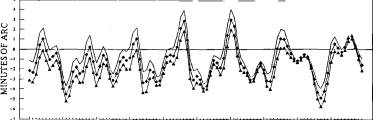sampling at 2-day intervals

_ SAROS 1_ SAROS 2_,_- SAROS 3

Fig. 4. Halley's Saros synchrony, depicting three sets of TMM error-patterns, over half-year periods, in identical phases of successive Saros cycles, for the years 1690, 1708 and 1726.

Halley made over 2000 meridian-transit lunar positional measurements, published posthumously, together with their GMT times. The `raw' data whose non-publication has been much remarked upon presumably com­prised the lunar limb positions, i.e. unadjusted by lunar semidiameter, which remain in his notebook (31). From his first five years of observations (1722-7) the lunar limb positions in right ascension were published, after which he switched to longitude of lunar centre, as more straightforward for checking of the theory. He did not publish declination or latitude values, as these were not essential for his purpose. I suggest that Halley's posthumous Astronomical Tables contained all the observational data that he wished to have published.

A check upon the error-values of a page of Halley's meridian-transit lunar ­centre longitude estimates, over June-August 1732, by comparing with values from the modern ILE program, gave a mean error of -14" ±20", a standard deviation of one-third of an arcmin. For the earlier-published limb observations given in right ascension, selecting January-April 1722, Bernard Yallop of the Nautical Almanac Office ascertained their accuracy as -10"+33". These were the first observations recorded using Greenwich Mean Time and the most accurate that anyone had then produced. For comparison, mean longitude errors in a set of Flamsteed's lunar limb meridian-transit readings Yallop found to be considerably larger, at 7"±70"" (32).

An evaluation of Halley's Saros method was performed by generating a set of TMM error-patterns, sampling at noon every two days over a six-month period, then repeating this procedure starting exactly one Saros later, and a third time for one Saros earlier. Superimposing these three sets of error ­patterns gave the error-curves shown in Fig. 4. The marvellous synchrony of the Saros appears in their concordance. A drift appears in values over these cycles due to a slight error in Newton's mean motion value. Correcting for this gave a still closer accord, well within an arcmin. Over the period for which Halley was using TMM, i.e. the 1720s and 30s, its mean motion had accumulated an error of 1-2 arcmin as can be seen in these diagrams (33). Halley claimed that his method was good enough for the longitude prize. It appears to have been the most accurate method of ascertaining lunar longitude produced in the first half of the eighteenth century.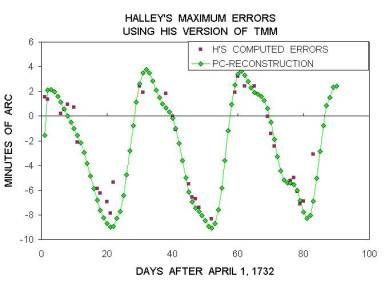FIG. 5. Comparison of error-patterns over a page of Halley's posthumously-published data, April-June 1733. His own error-estimates, in arcmin of zodiacal longitude, are compared with the computer replica. Maximal errors here arise at 30-day intervals.

None of Halley's contemporaries could check Halley's new method however, as no-one but he had the data! His dog-in-a-manger attitude towards publication of his data earned him a stern rebuke from the ageing Newton as President of the Royal Society in 1725 (34). Halley was in this respect his own worst enemy: by the time his data were published posthumously in 1749, more accurate lunar theories were making his approach, based upon the Horroxian theory of Newton, obsolete.Halley's normally communicative temper greatly failed him in this respect. Historians have tended to misunderstand what Halley was doing in his two decades as Astronomer Royal, little appreciating that he was developing a method of error-correction of Newton's method based on the Saros.

In the 1730s, Halley found that his lunar theory was periodically yielding errors of up to eight minutes of arc - fully twice what he had averred to the Royal Society as the maximum generated by Newton's theory (35). Figure 5 shows the worst period, comparing the computer model of Halley's version of TMM with the error values Halley was faithfully recording in his tables. Halley had devised a modified version of Newton's lunar theory, altering the sequence of equations and omitting the seventh equation, as it made his method slightly less accurate: the errors shown in Fig. 5 are larger than those which would be generated by TMM. We can only guess how Halley then felt, but he faithfully recorded these errors, as were required for his Saros method. The overall accuracy of his observational procedure as within an arcmin appears from comparing his error-values with the computer's graph.

Halley was far from being the only astronomer to publish tables based upon Newton's lunar theory, however the names of others are today hardly remembered: Charles Brent (London), Robert Wright (Manchester), Charles Leadbetter (London), Richard Dunthorne (Cambridge), Pierre LeMonnier (Paris), Peter Horrebov (Uppsala), Nicholas Grammaticus (Ingolstadt) and Angelo Capello (Venice). Nicholas Delisle in Paris also composed such tables but never printed them. My researches have generally confirmed the view expressed by Craig Waff in 1973 that: `nearly all new tables constructed during the first half of the eighteenth century utilized in some fashion his [Newton's] tabular theory' (36).

The `theory' published as TMM passed into a swift oblivion once the researches of Clairaut and others using the differential calculus had derived a lunar theory which really was based upon gravity theory. Clairaut's Theoriae motus lunae appeared in 1753. This Continental achievement entered Britain in the shape of Tobias Meyer's tables (37), which finally won the longitude prize in I76o, and which Maskelyne used to construct the Nautical Almanac, giving predicted lunar longitudes in apparent time with errors generally less than half an arcmin.

7 CONCLUSION

A new dimension in the history of astronomy has appeared in recent decades, as home computers have become able to recreate the conditions of ancient skies to an accuracy surpassing that available for historical astronomers. For the seventeenth and eighteenth centuries, this modern approach is associated with the names of Owen Gingerich and Curtis Wilson in the USA. It has here been applied to Newton's 1702 lunar theory, viewing the latter as a mechanism able to generate five positions in celestial longitude and one in latitude for any given time. While this theory was an improvement on its predecessors, it did not reach the accuracy which Newton, Halley or Gregory ascribed to it. It at least doubled the number of tables required on account of its extra equations, which is doubtless why it took over two decades before sets of tables based upon TMM began to be published. Our ability to assess the relevance of its separate components was limited by the absence of modern analogies to its steps of equation, its rocking apse and its amplitude-modulating functions. Halley's insight into the workings of the Saros cycle gave him a potentially effective empirical method for estimating the errors generated by TMM, though neither Continental nor British astronomers adopted it.

While the Newtonian procedure for finding lunar longitude was a substantial improvement on earlier methods, it had hardly been deduced from a gravity theory (38). Astronomers in the eighteenth century would sometimes see its accuracy as a testimony to such, as was clearly implied in the Principia of 1713 (39). On the matter of its linkage to gravity theory, French astronomers tended to remain more sceptical than the English (40). If Newton's 1702 lunar theory had to any degree been derived from a gravity theory, then that linkage was intuitive rather than deductive.

APPENDIX: WORKS UTILIZING TMM

Nicholas Delisle Tables du soled & de la lune (Paris Observatory archives), 1716; Peter Horrebov Nova Theoria Lunae (Uppsala archives), 1718; Nicasius Grammaticus Tabulae Lunares (Ingolstadt), 1726; Robert Wright New & Correct Tables of the lunar motions, 1732; Angelo Capello Astrosophiae Numericae, 1738; Charles Leadbetter Uranoscopia 1735; Richard Dunthorne Practical Astronomy of the Moon, 1739; Charles Brent The Compendious Astronomer, 1741; Pierre leMonnier Institutions Astronomiques, 1746; Edmond Halley Tabulae Astronomicae, 1749.

REFERENCES

(i) Forbes E., 1975. Greenwich Observatory is Origins and Early History, London: Taylor & Francis.

(2) Kollerstrom, N. & Yallop, B., Flamsteed's Lunar Data, 1692-95, sent to Newton, Journal for the History of Astronomy xxvi (1995) 237-246. The table of 16 meridian-transit lunar limb observations plus error estimates sent by Flamsteed to Newton in February 1695 (The Correspondence of Isaac Newton, IV, 1967, 85), may be all that remains of the data Newton and Gregory were shown at Greenwich.

(3) Howse, D., 1994. Longitude-finding by Astronomy, Companion Encyclopaedia of the

History and Philosophy of the Mathematical Sciences, I. Grattan-Guinness ed., II, 113-8.

(4) Horrox's theory was published in Opera Posthuma ed. by Wallis, J., 1673. Flamsteed's version was given in his De Sphaera in Jonas Moore's A New System of the Mathematics, 1681, 1, 1-75.

(5) A letter from Mr Flamsteed, 1683. Phil. Trans., 13, 404-8.

(6) Wilson C., Predictive astronomy in the century after Kepler 1989. The General History of Astronomy, ed, R. Taton & C. Wilson, CUP Cambridge, 197-201. (7) Op. cit. (E), 63-65.

(8) It was removed in Euler's Theoria motuum Lunae of 1753: Forbes, E., 1980. Tobias Mayer (1723-62) Pioneer of Enlightened Science in Germany, 143.

(9) Letter to Flamsteed of 20 July 1695, 1967. Correspondence IV, 151.

(10) Howse, D., I98o. Greenwich Time and the Discovery of Longitude, Oxford, 51.

(11) Gaythorpe, S.B., 1925. On Horrock's Treatment of the Evection and the Equation of the Centre, Mon. Not. R. astr. Soc., 85, 858-865.

(12) Whiteside, D.T., 1976. Newton's Lunar Theory: From High Hope to Disenchantment Vistas in Astronomy 19, 317-28.

(13) Kollerstrom, N., 1991. Newton's Two Moon-Tests Brit. J. Hist. Science 24, 369-72. (I4) Memoranda by Gregory, 1698, Correspondence, IV 277; Brewster, D. Memoirs of Isaac Newton 1855, 1, 313.

(15) A Memorandum by Flamsteed, 1695, Correspondence, IV, 135.

(16) Gregory, D., 1702. Astronomiae Physicae et Geometricae Elementa Oxford, 332-336; trans. The Elements of Physical and Geometrical Astronomy, 1715; I.B.Cohen, 1975. Newton's Theory of the Moon's Motion, Folkstone.

(17) Whiteside, op. cit. (12) 324.

(18) Wilson, op. cit. (6) 267.

(19) Gingerich, O. & Welther, B., 1974. Note on Flamsteed's Lunar Tables, Brit. J. Hist. Science, 7,257-8.

(20) Cohen, op. cit. (I6), 111.

(21) Roy, A.E., 1978. Orbital Motion 6th Edn., 87; 9 = 2Esin(A-M)-I 25E2sin2(A-M) where E is eccentricity and (A - M) is mean anomaly.

(22) A `correct' use of TMM, i.e. with sign of its 6th equation reversed, was published in 1710 by the Rev. H.Cressner: An Account of the Moon's Eclipse ... compared with Calculation, Phil. Trans. 27, 1710-1712, 16-19.

(23) Halley, E., 1732. A Proposal of a Method for Finding Longitude at Sea within a Degree, Phil. Trans., 37, 185-195, 191.

(24) Letter of Flamsteed to Caswell, 1703, in Baily, F., 1835. An Account of the Revd. John Flamsteed, p. 213.

(25) Improved Lunar Ephemeris (Washington, 1954); with improvements to ILE published in The Astronomical Ephemeris (Washington and London, 1972).

(26) Motte trans. of the Principia, II University of California Press, 1962, 473-477. (27) Wilson, op. cit. (6) pp. 162, 201, 267-8.

(28) Neugebauer, O., 1957. The Exact Sciences in Antiquity Brown U.P., 141-2. Nicholas Delisle affirmed in 1750 that `M. Halley avoit etabli cette periode de 223 lunations...', adding that Halley had in 1714 `chosen' the Saros that began in 1700 to compute solar and lunar eclipses. Lettre sur les Tables Astronomiques de M.Halley, Archives of the Paris Observatory. Shortly after, D'Alembert referred to ' Le periode de M.Halley' (Recherches sur differens points importants de Systeme du Monde, Paris 1754, 3, xv).

(29) Cotter, C. H., 1968. A History of Nautical Astronomy, 200-1; Forbes op. cit. (1), 73-87.

(30) Baily, F., 1835. Account of the Astronomical Observations of Dr Halley Memoirs of the Royal Astronomical Society, VIII, 169-19o, 189.

(3I) Halley, Astronomical Notebook, manuscript copy, 518 pp., RAS Library.

(32) Flamsteed's letter to Newton, 7 Feb. 1695 Correspondence, IV, 85; Kollerstrom & Yallop, op. cit (2).

(33) D'Alembert inferred an error in Halley's mean motion value from his recorded error­values, of 2'10": op. Cit. (28), III, 34-39.

(34) Baily, F., op. cit. (24), 188.

(35) Wilson, op. cit. (6), 162.

(36) Quoted by I.B.Cohen, op. cit. (i6), 79.

(37) Leonhardt Euler's Theoriae Motuum Lunae of 1753 generated errors of ± 5 arcminutes, i.e. was hardly more accurate than Newton's: Forbes, op. cit. (8), 136-145.

(38) Certain seasonal modulations present in TMM were later claimed as derivable from gravity theory, viz the second and fifth lunar equations had their peak amplitudes vary seasonally, inversely as the cube of Sun-Earth distance i.e. ±4.8%; also, the two new annual equations.

(39) Whewell, W., 1837. History of the Inductive Sciences, II, 209-10.

(40) M.Bailly, 1779. Histoire de l’Astronomie Moderne, 3, 150. See also, Craig Waff's Newton and the Motion of the Moon, An Essay Review, Centaurus 1977, 21, 64-75, 68.